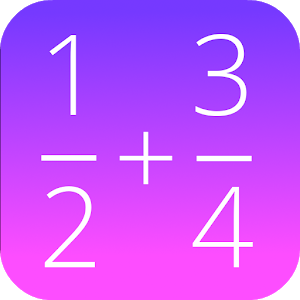Français

Chaque jour nous offrons des appli et jeux Androïdes GRATUITES que vous devrez autrement acheter.\$0.99
EXPIRÉ

# Android Giveaway of the Day - Fractions Math Pro

This math app is a fractions calculator with various functions!
\$0.99 EXPIRÉ
Note des utilisateurs: 6

This giveaway offer has been expired. Fractions Math Pro is now available on the regular basis.

This calculator is the pro version of 'Fractions Math', and completely without advertisement.

This math app is a fractions calculator with various functions:

- add, subtract, multiply and divide fractions.
- convert fractions in decimals,
- simplify fractions
- compare fractions
- find the lowest common denominator
- fraction trainer, to learn fraction calculations

GK Apps

Education

4.0

279k

Everyone

1.6 and up

## Commentaires sur Fractions Math Pro

Thank you for voting!